# A Pound of Quarters vs. a Pound of Dimes: Which Is Heavier, and Which Is Worth More?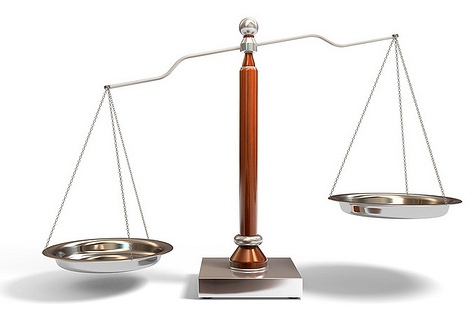Many youngsters have heard, and perhaps even fallen for, the classic teaser: "Which is heavier: a ton of bricks or a ton of feathers?"

The juxstaposition of the words "brick" and "feather," in the context of weight, serves as perfect misdirection. It catches a lot of people out the first time they encounter it.

Yet if we want to get really picky, the answer is trickier than it seems at first (OK, second) sight: The sheer bulk of a mountain of feathers is hard to get on any balance to compare with a house of bricks. The two proposed collections of objects are, in a very real sense, incomparable. Moreover, it seems unlikely that anybody has ever amassed the required tonnage of feathers.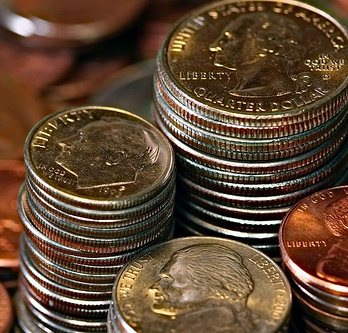Closer to earth, and ignoring gravitational variations due to extreme volume variation, we pose the following two questions relating to U.S. coinage:
1. Which is heavier: a pound of quarters or a pound of dimes?
2. Which is worth more: a pound of quarters or a pound of dimes?

The first one seems unambiguous and straightforward, right? Bear in mind, however, the difficulty one experiences in real life when trying to purchase exactly one pound of bananas or peaches.

Presumably the second one can be settled if we can get our hands on the relevant figures. It certainly isn't an apples-and-oranges comparison.

If you're feeling brave, you could use these questions as the basis for a bar bet or two, perhaps wagering \$20 on the correct outcome. But read to the bitter end here first, so you'll be well-prepared to argue your case if the need arises.

Let's have the U.S. Mint weigh in with key information about the coins in question.

According to their coin specification webpage, a dime weighs 2.268 grams, and a quarter weighs 5.670 grams.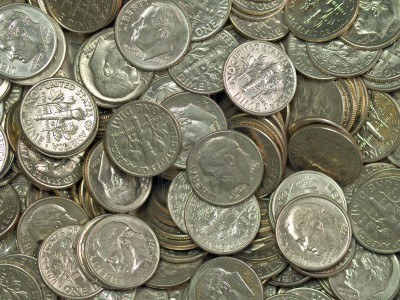(OK, technically, that's just for brand-new, shiny, "legal tender coins presently in production for United States Mint Annual Sets." Who knows what's in circulation from previous releases, or what state the coins in our pockets are in. We're going to work in good faith with the Mint data. If you seek inspiration, try this video of nickel-plated quarters being struck at the U.S. Mint in Philadelphia.)

Let's focus on one dime at a time. According to Wolfram Alpha, a single gram is 0.002205 pounds, or about the weight of a dollar bill (or paper clip), from which it follows by multiplication that 2.268 grams is 0.00500094 pounds.

Curiously, as we go to press, Wolfram Alpha gives a more rounded 0.0050001 pounds when asked that directly. (Less accuracy on a larger scale? That's odd.)

It's also noted there that this is 91 percent of the weight of a penny (a penny weights 2.500 grams), but we saw no mention of the fact that it's the weight of a dime.

Based on the first calculation, a pound of dimes seems to contain 199.9624070 dimes (we just divided 0.00500094 into 1). That's close to 200 dimes, which is exactly \$20. So would 200 dimes actually balance a 1-pound weight exactly on a scale, if push came to shove?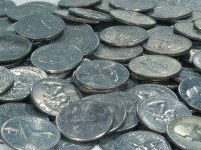Is the 199.9624070 vs. 200 mismatch the result of a less-than-perfect metric-to-pound conversion?

Meanwhile, Wolfram Alpha converts the Mint figure for the weight of a quarter to 0.0125 pounds, and also records that this is 1.00002 times the mass of a quarter. Whoa! This suggests that a Mint quarter is heavier than what Wolfram Alpha thinks a quarter weighs.

Had we gone with 5.670 times 0.002205, we'd have obtained a more precise (but is it more accurate?) 0.0125023 pounds. Wolfram Alpha appears to think that a quarter has a mass of 5.7 grams, which is strange, as that's noticeably heavier than a Mint quarter.

If we go with the 0.0125-pounds-per-quarter conversion, then a pound of dimes contains 1/0.0125 = 80 quarters, which is exactly \$20. However, if we go with the 0.0125023-pounds-per-quarter conversion, then a pound of quarters contains 1/0.0125023 = 79.985282708 quarters, which comes out to \$19.996320677.

The first surprise is that a pound of dimes and a pound of quarters are essentially worth the same amount of money, namely \$20, a coincidence on two levels that isn't so well-known.

The value of large quantities of dimes and quarters, either individually or mixed, can thus be assessed using the same conversion factor. Banks, of course, have known about this "silver" standard all along.

Moreover, isn't it remarkable that 1 pound of these coins is worth \$20? Magic or coincidence? Keep reading.

Let's take a closer look. It appears that 200 dimes slightly overshoots a pound, so if there is a strictly enforced weight limit of 1 pound, then you'll have to settle for 199 dimes, which is only \$19.90. Similarly, it seems that 80 quarters either weighs exactly a pound or overshoots it. Again, if there is a strictly enforced weight limit of 1 pound, then you'll have to settle for 79 or 80 quarters, depending on which conversion you believe is accurate. That comes out to either \$19.75 or \$20.

So if Question 2 above really meant "which is worth more: the contents of a jar of quarters or the contents of a jar of dimes, given a weight limit of one pound?" then in one sense it could go either way.

On the other hand, if somebody asked you for a pound of dimes, they'd be disappointed if you shortchanged them. So if Question 2 really meant "which is worth more: the contents of a jar of quarters or the contents of a jar of dimes, each of which must weigh at least one pound?" then, well, it also gets confusing!

You should be starting to wonder if there is such a thing as an exact pound of dimes (or quarters), in the sense that a collection of one of these coins could perfectly balance a pound weight on a balance.

Some readers may wish to redo the above estimates using more accurate conversion factors, in the hope that more definitive conclusions can be reached.

Let's return to the figures given by the Mint for the dime (2.268 grams) and quarter (5.670 grams), leaving aside for now the issue of exactly what 1 pound of such coins would consist of.

Multiplying each number by 1,000, we conclude that a dime weighs 2,268 milligrams and a quarter weighs 5,670 milligrams. No obvious relationship between those numbers jumps out at us, but let's factor them into primes:

First note that 2,268 = 2 x 2 x 3 x 3 x 3 x 3 x 7, and 5,670 = 2 x 3 x 3 x 3 x 3 x 5 x 7. It follows, by using the highest visible power of each prime factor, that 2 x 2 x 3 x 3 x 3 x 3 x 5 x 7 = 11,340 is a multiple of both. In fact, it's their least common multiple. Even better, we have 11,340 = 5 x 2,268 = 2 x 5,670.

We've just discovered, with the help of prime numbers and the U.S. Mint specifications, something that was in front of us all along: five new dimes weigh the exact same as two new quarters! Of that there can be no doubt. Pound for pound, half dollar for half dollar, they come out the same. Well, the second part of that is certainly true.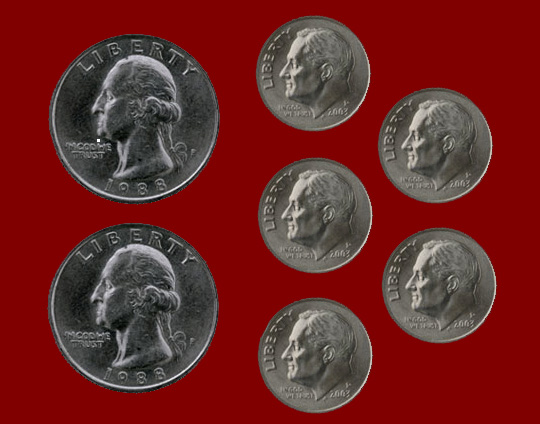(The next time you ask for change of a dollar in a store, ask for seven coins with the property that some of them will exactly balance all the others, and see what kind of response you get. )

The desired weight balance in this case follows from splitting the dollar's worth in the adjacent photo right down the middle. Well, maybe for true balance there we need to aim for a little left of center.

It turns out that either of those collections (the dimes or the quarters) can indeed be replaced with a half-dollar. Thirteen years ago somebody asked Snopes, "Is is true that in U.S. currency, a pound of dimes, quarters, and half-dollars in any combination is worth \$20?" One respondent opined that the answer is yes, because "they are all worth \$1 per 22.68g." Rivkah Chaya shed some light on why: "It works, because back when dimes, quarters, half-dollars, and dollar coins were all silver, they contained their respective face values of silver, so a quarter would weigh 2.5 x what a dime did." Hindsight is 20/20.

Fair enough, it's all by design, not accident -- presumably also the bit about them weighing 1 pound in suitably rounded quantities.

But isn't it then remarkable that the weights (in milligrams) forced on individual dimes and quarters comes out to be 1) whole numbers, and 2) numbers with no prime factors larger than 7? Or is that just a convenient near-coincidence due to (here comes that word one more time) rounding?

To return to the two questions we posed at the outset, the answers appear to be the same. As in, "They're the same!"

Here's a final question to ponder: "Which is greater: a pound's worth of quarters or a pound's worth of dimes?"

You may wish to consult the Bank of England before jumping to any conclusions. If we are speaking of Sterling currency, then the answer is unlikely to be the same (i.e., "the same"). Remember the fruit quandary we mentioned above; it probably plays a role here.

Thanks once again to Spelman College's Dan Bascelli for graphics production and assistance. Thanks also to Dave Aton and Bruce Reznick for helpful pointers and discussions.